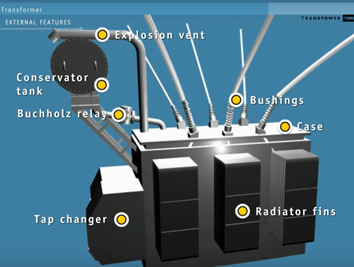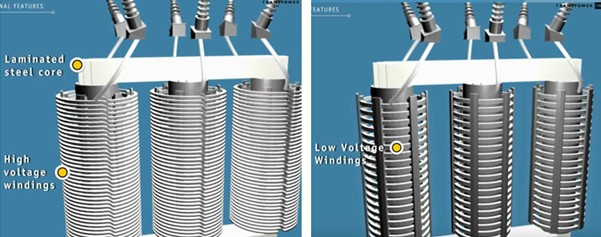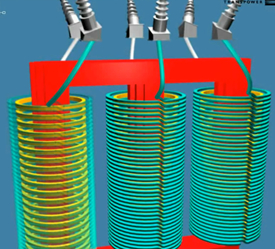# Introduction to transformer: How it works?

### The transformer is used to convert the incoming voltage at the location to the correct voltage (for the submersible motor in case of ESP). Transformer selection is based on mainly 4 parameters:

• Power rating in KVA (Kilo Volt Amperes),
• Primary voltage,
• Secondary voltage,
• Tap arrangement.

### Power rating in KVA for three phase transformer:

The calculation of power rating in KVA for a Three Phase Transformer is based on Winding Voltage and Amperage information. The simple formula to calculate the rating of three phase transformers is:

KVA = (√3. V x I) /1000

Refer to the post titled “How to Calculate the Required KVA Rating for three Phase Transformers? ” for more details.

### Step-Up and Step-down Transformer:

Transformers are composed of a magnetic path (iron core ring) wrapped in two coils. These transformers are generally an isolation type having no electrical connection between its two coils.

One coil is connected to an AC input voltage and is called the primary coil. The other coil is connected to an output circuit with a load resistance and is called the secondary coil.

Transformers can either step-up or step-down a voltage. In Step-Down Transformer, The number of turns (N1) in the primary coil is greater than the number of turns (N2) in the secondary coil. In Step-Up Transformer, the number of turns (N2) in the secondary coil is greater than the number of turns (N1) in the primary coil.

### Transformer Transformation Ratio:

The ratio of the number of turns (N2/N1) in the secondary winding to the number of turns in the primary winding (if leakage flux is neglected) is a characteristic of the transformer, named Transformation Ratio (K). Transformation Ratio is also equal to the ratio of secondary voltage to the primary voltage.

• If N2>N1 i.e. K>1, then the transformer is called step-up transformer (V2>V1).
• If N2<N1 i.e. K<1, then the transformer is called step-down transformer (V2<V1).

### Key components of a transformer:

On the outside of the transformer are a number of external components: some help to remain at a good working temperature, some help make it safer in the event of an accident, and some are used for monitoring.• The radiator fins help cool the oil.
• The conservator tank allows for the oil to expand and contract as it heats and cools.
• The explosion vent protects the transformer in the event of a major fault.
• The bushings insulate the conductors within into the case.
• The tape changer fits the exact amount of voltage change.
• The Buchholz relay detects the presence of gas caused by the deterioration of the oil and/or a rapid increase in oil pressure.

Inside the case at the transformer, there are three actual voltage transformers, one for each phase. Each transformer is a laminated steel core surrounded by two different layers of wire windings.Let’s see how a step-down transformer works, the current comes into the transformer through a conductor that joins onto the high-voltage windings. This induces a magnetic flux in the iron core. This flux induces a current in the low voltage windings.Because there are fewer complete loops of wire in the second set of windings (step-down transformer), the current sent out from them has a lower voltage than the one that came in. To adjust the final voltage further, we can use tape changes. These, reduce the number of windings on the low voltage side still further by changing the location of the conductor that leads out of the transformer.

### You May Also Like…